Home / Blog / Data Science / Data Science Formulae

# Data Science Formulae

• September 29, 2023
• 4191
• 85### Meet the Author : Mr. Bharani Kumar

Bharani Kumar Depuru is a well known IT personality from Hyderabad. He is the Founder and Director of Innodatatics Pvt Ltd and 360DigiTMG. Bharani Kumar is an IIT and ISB alumni with more than 17 years of experience, he held prominent positions in the IT elites like HSBC, ITC Infotech, Infosys, and Deloitte. He is a prevalent IT consultant specializing in Industrial Revolution 4.0 implementation, Data Analytics practice setup, Artificial Intelligence, Big Data Analytics, Industrial IoT, Business Intelligence and Business Management. Bharani Kumar is also the chief trainer at 360DigiTMG with more than Ten years of experience and has been making the IT transition journey easy for his students. 360DigiTMG is at the forefront of delivering quality education, thereby bridging the gap between academia and industry.

## Measures of Central Tendency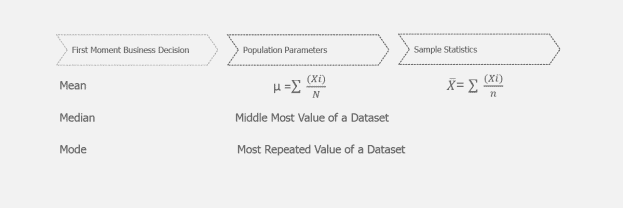## Measures of Dispersion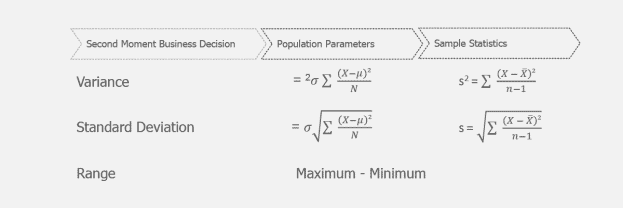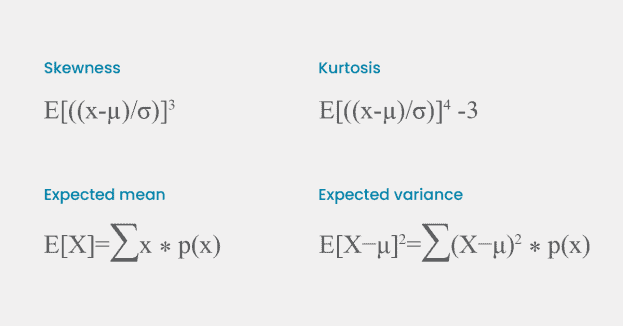360DigiTMG also offers the Data Science Course in Hyderabad to start a better career. Enroll now!

## Graphical Representation

Box Plot calculations

Upper limit = Q3 + 1.5(IQR)
IQR: Q3 – Q1
Lower limit = Q1 – 1.5(IQR)

8) Histogram calculations

Number of Bins = √n

Where n: number of records
Bin width = Range / Number of bins
Where Range: Max – Min value
Number of bins: √number of records

NormalizationStandardizationRobust Scaling12) Theoretical quantiles in Q-Q plot = X - µ / σ

Want to learn more about data science? Enroll in this Data Science Classes in Bangalore to do so.

Where X: the observations
µ: mean of the observations
σ: standard deviation

13) Correlation (X, Y)

r = Σ((Xᵢ - X̄) * (Yᵢ - Ȳ)) / √(Σ(Xᵢ - X̄)² * Σ(Yᵢ - Ȳ)²)

Where:
Xᵢ and Yᵢ are the individual data points for the respective variables.
X̄ (X-bar) and Ȳ (Y-bar) are the sample means of variables X and Y, respectively.
Σ represents the sum across all data points.

14) Covariance (X, Y)

Cov(X, Y) = Σ((Xᵢ - X̄) * (Yᵢ - Ȳ)) / (n - 1)

Where:
Xᵢ and Yᵢ are the individual data points for the respective variables.
X̄ (X-bar) and Ȳ (Y-bar) are the sample means of variables X and Y, respectively.
Σ represents the sum across all data points.
n is the total number of data points.

Are you looking to become a Data Scientist? Go through 360DigiTMG's Data Science Course in Chennai

Box-Cox TransformationYeo- Johnson Transformation## Unsupervised Techniques

Clustering

Distance formulae(Numeric)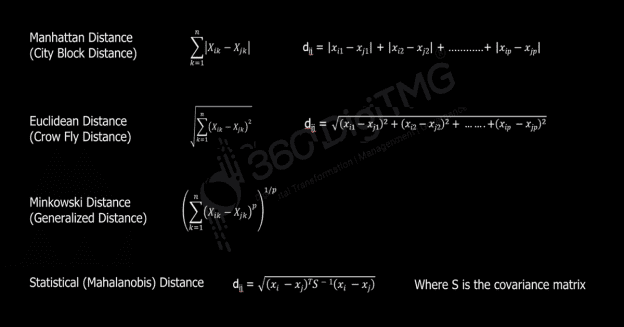Distance formulae (Non- Numeric)Dimension Reduction

Also, check this Data Science Course Training in Hyderabad to start a career in Data Science.

Singular Value Decomposition (SVD)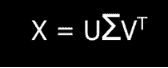## Association Rule

Support (s):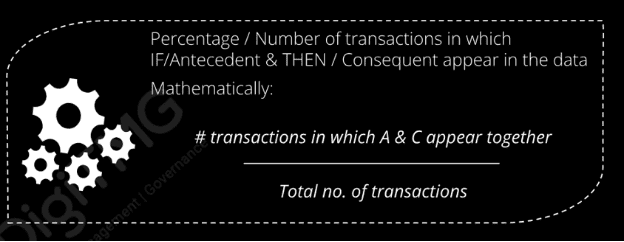Confidence (c)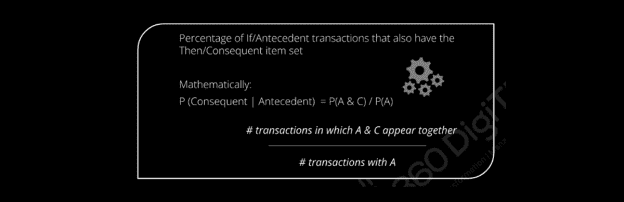Lift (l)## Recommendation Engine

Cosine Similarity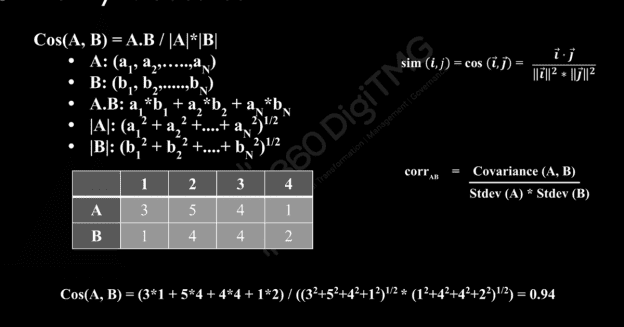Network Analytics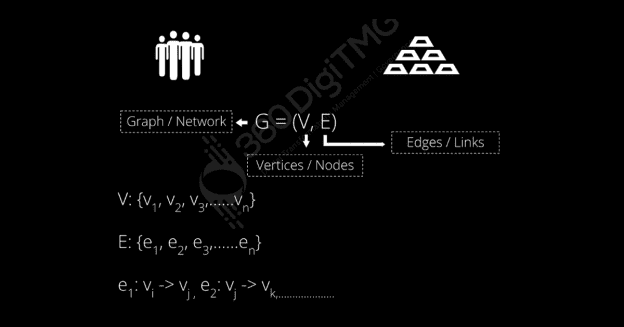Closeness CentralityBetweeness Centrality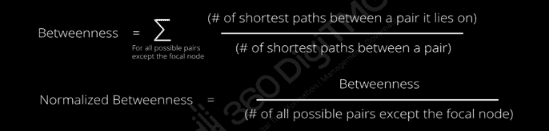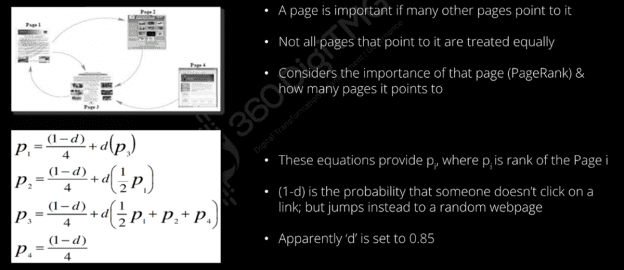## Text mining

Term Frequency (TF)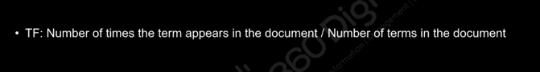Inverse Document Frequency (IDF)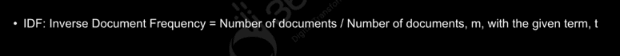TF-IDF (Term Frequency-Inverse Document Frequency)## Supervised Techniques

Bayes' Theorem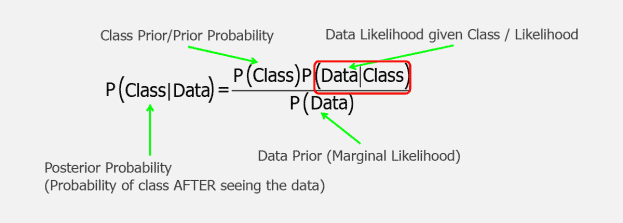K-Nearest Neighbor (KNN)

Euclidean distance is specified by the following formula,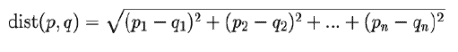Decision Tree:

Information Gain = Entropy before – Entropy after

EntropyConfidence Interval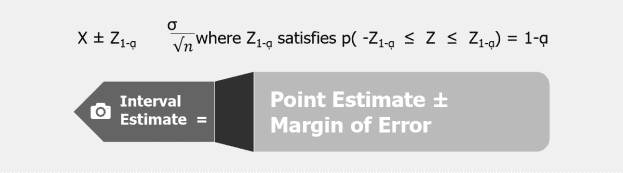### Regression

Simple linear Regression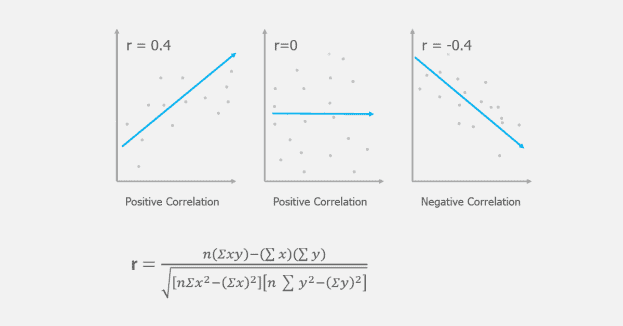Equation of a Straight Line

The equation that represents how an independent variable is related to a dependent variable and an error term is a regression model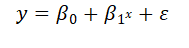Where, β0 and β1 are called parameters of the model,

ε is a random variable called error term.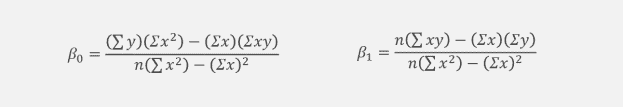## Regression Analysis

R-squared-also known as Coefficient of determination, represents the % variation in output (dependent variable) explained by input variables/s or Percentage of response variable variation that is explained by its relationship with one or more predictor variables

• Higher the R^2, the better the model fits your data
• R^2 is always between 0 and 100%
• R squared is between 0.65 and 0.8 => Moderate correlation
• R squared in greater than 0.8 => Strong correlation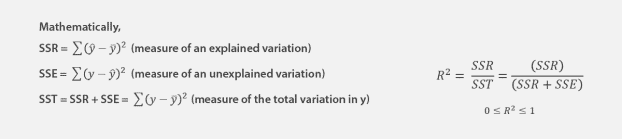Multilinear Regression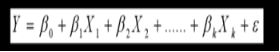Logistic Regression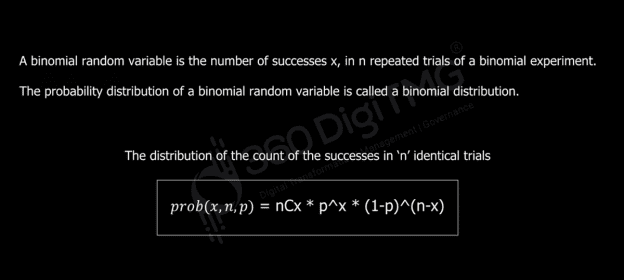Lasso and Ridge Regression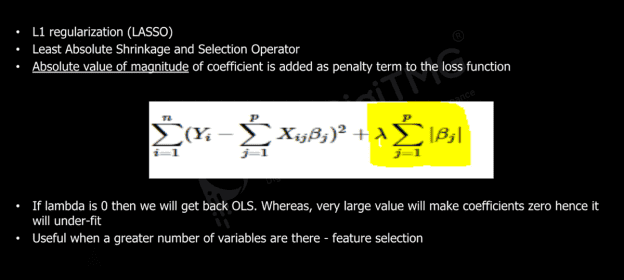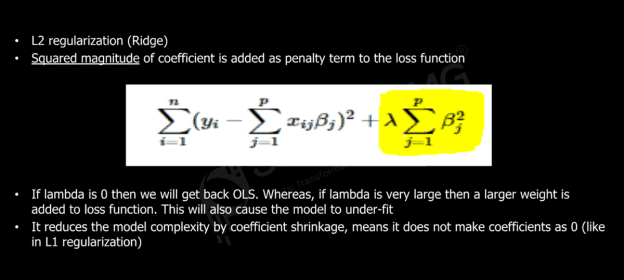Residual Sum of Squares + λ * (Sum of the absolute value of the magnitude of coefficients)

Where, λ: the amount of shrinkage.

λ = 0 implies all features are considered and it is equivalent to the linear regression where only the residual sum of squares is considered to build a predictive model

λ = ∞ implies no feature is considered i.e., as λ closes to infinity it eliminates more and more features

• Ridge = Residual Sum of Squares + λ * (Sum of the squared value of the magnitude of coefficients)

Where, λ: the amount of shrinkageNegative Binomial Distribution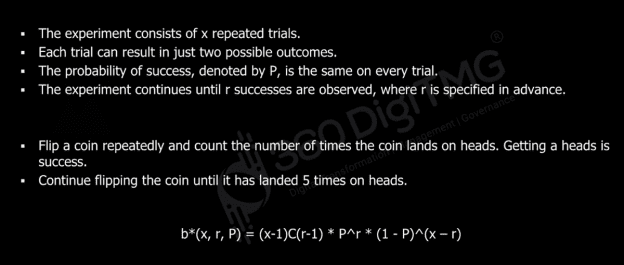Poisson Distribution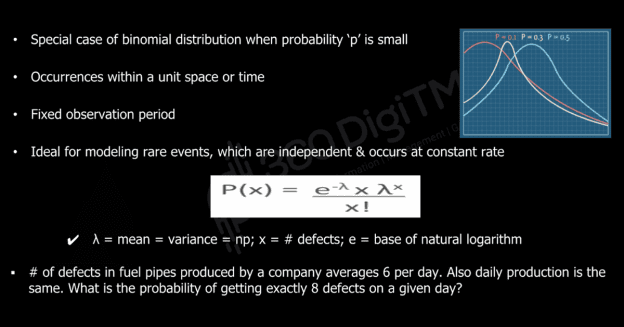Become a Data Scientist with 360DigiTMG Best Institute for Data Science Course in Chennai. Get trained by the alumni from IIT, IIM, and ISB.

### Time Series:

Moving Average (MA)

The moving average at time "t" is calculated by taking the average of the previous "n" observations:

MAₜ = (yₜ + yₜ₋₁ + yₜ₋₂ + ... + yₜ₋ₙ) / n

• Exponential Smoothing

Exponential smoothing gives more weight to recent observations. The smoothed value at time "t" is calculated using a weighted average:

Sₜ = α * yₜ + (1 - α) * Sₜ₋₁

Where "α" is the smoothing factor.

• Autocorrelation Function (ACF)

Correlation between a variable and its lagged version (one time-step or more)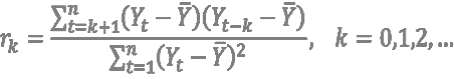Yt = Observation in time period t
Yt-k = Observation in time period t – k
Ӯ = Mean of the values of the series
rk = Autocorrelation coefficient for k-step lag

• Partial Autocorrelation Function (PACF):

The partial autocorrelation function measures the correlation between observations at different lags while accounting for intermediate lags. The PACF at lag "k" is calculated as the coefficient of the lag "k" term in the autoregressive model of order "k":
PACFₖ = Cov(yₜ, yₜ₋ₖ | yₜ₋₁, yₜ₋₂, ..., yₜ₋ₖ₋₁) / Var(yₜ)

### Confusion Matrix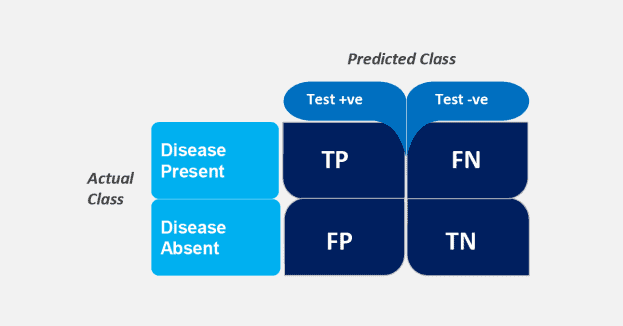• True Positive (TP) = Patient with disease is told that he/she has disease
• True Negative (TN) = Patient with no disease is told that he/she does not have disease
• False Negative (FN) = Patient with disease is told that he/she does not have disease
• False Positive (FP) = Patient with no disease is told that he/she has disease

Overall error rate = (FN+FP) / (TP+FN+FP+TN)

Accuracy = 1 – Overall error rate OR (TP+TN) / (TP+FN+FP+TN); Accuracy should be > % of majority class

Precision = TP/(TP+FP) = TP/Predicted Positive = Prob. of correctly identifying a random patient with disease as having disease

Sensitivity (Recall or Hit Rate or True Positive Rate) = TP/(TP+FN) = TP/Actual Positive = Proportion of people with disease who are correctly identified as having disease

Specificity (True negative rate) = TN/(TN+FP) = Proportion of people with no disease being characterized as not having disease

• FP rate (Alpha or type I error) = 1 – Specificity
• FN rate (Beta or type II error) = 1 – Sensitivity
• F1 = 2 * ((Precision * Recall) / (Precision + Recall))
• F1: 1 to 0 & defines a measure that balances precision & recall

Forecasting Error Measures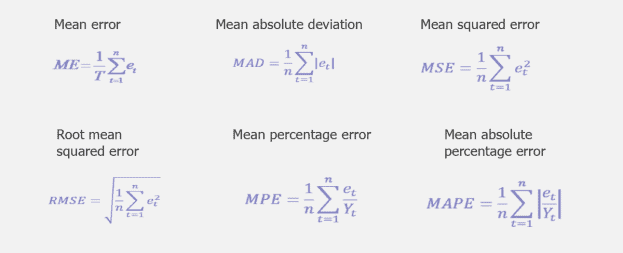• MSE = (1/n) * Σ(Actual – Forecast)2
Where n: sample size
Actual: the actual data value
Forecast: the predicted data value
• MAE = (1/n) * Σ |Actual – Forecast| Where n: sample size
Actual: the actual data value
Forecast: the predicted data value
• MAPE = (1/n) * Σ |Actual – Forecast| / Actual
Where n: sample size
Actual: the actual data value
Forecast: the predicted data value
• RMSE = √(1/n) * Σ(Actual – Forecast)2
Where n: sample size
Actual: the actual data value
Forecast: the predicted data value
• MAD = (1/n) * Σ |Actual – µ|
Where n: sample size
Actual: the actual data value & µ: mean of the given set of data
• SMAPE = (1 / n) * Σ( |Fᵢ - Aᵢ| / (|Fᵢ| + |Aᵢ|) ) * 100%
Where:
Fᵢ represents the forecasted value.
Aᵢ represents the actual value.

Looking forward to becoming a Data Scientist? Check out the Professional Course of Data Science Course in Bangalore and get certified today.

### Data Analyst Courses in Other Locations